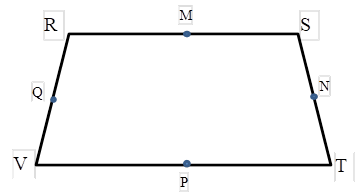Chapter 4.4, Problem 9EElementary Geometry For College St...

7th Edition
Alexander + 2 others
ISBN: 9781337614085

Solutions

Chapter
SectionElementary Geometry For College St...

7th Edition
Alexander + 2 others
ISBN: 9781337614085
Textbook Problem

For Exercises 9 and 10, consider isosceles trapezoid RSTV with R S ¯ | | V T ¯ , and midpoints M, N, P, and Q of the sides.Would RSTV have symmetry with respect to a) M P ↔ ? b) Q N ↔ ?Exercises 9, 10

To determine

a)

To find:

The symmetry of the trapezoid RSTV, with respect to MP.

Explanation

Consider the following isosceles trapezoid RSTV.

In an isosceles trapezoid, RS¯||VT¯.

M is the midpoint of the side RS¯ and P is the midpoint of the side VT¯

To determine

b)

To find:

The symmetry of the trapezoid RSTV, with respect to QN.

Still sussing out bartleby?

Check out a sample textbook solution.

See a sample solution

The Solution to Your Study Problems

Bartleby provides explanations to thousands of textbook problems written by our experts, many with advanced degrees!

Get Started

In Problems 21-24, if , find each of the following using the properties of logarithms. 24.

Mathematical Applications for the Management, Life, and Social Sciences

Rewrite the expression without using the absolute-value symbol. 5. |55|

Single Variable Calculus: Early Transcendentals, Volume I

Evaluate 1(x41+x6)2dx.

Single Variable Calculus: Early Transcendentals

True or False: converges.

Study Guide for Stewart's Multivariable Calculus, 8th

Which is the best graph of r = 1 − sin θ for 0 ≤ θ ≤ π?

Study Guide for Stewart's Single Variable Calculus: Early Transcendentals, 8th